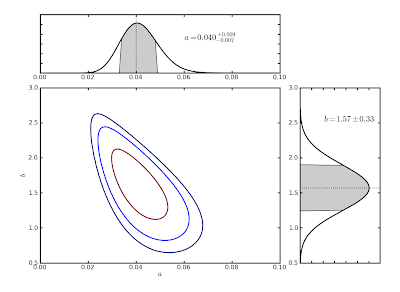## Friday, October 15, 2010

### Programming Investment: Making Figures

If you continue on in astronomy, you'll find (if you haven't already) that most of the actual time you spend doing research is, in fact, programming. Not just programming to actually do whatever analysis you're doing, but often a comparable amount of time putting together nifty professional-looking, presentable figures to communicate your results.

With this in mind, you should be thinking about building an accessible toolkit of useful routines that perform tasks that you expect to repeat often. A first example of something like this would be the function we had you write a few weeks ago that calculates a 1-D confidence interval, given vectors of x and p(x). You should consider writing similar routines for common plotting tasks.

For example, I've written a function called "plot_posterior" that takes as input x and p(x) (or L(x)--not necessarily normalized), just like your confidence interval function, and makes a pretty plot, as follows:

>>> x = arange(-2,6,0.001) #make x vector
>>> px = normal(x,2,1) #evaluate N(2,1) on x
>>> plot_posterior(x,px,name='x',labelpos=(0.65,0.8)) #make the plot
(Note the default thicker-than-normal axes and lines, which is the quickest way to make your plots look more presentable and should become a habit.)

The function also has other optional parameters, such as plotting different confidence intervals, marking the median value instead of the mode as the representative value, using a symmetric interval instead of the shortest interval, turn off shading, turn off labeling, change the number of decimal places to which the result and errors are quoted, etc. This way, whenever I want to make a nice plot of a posterior distribution with regions marked and labeled and all, I don't have to start from scratch.

I've also written a similar function ('plot_posterior2d') to summarize the results of a two-dimensional parameter estimation, as you are currently working on with the Fischer & Valenti data. This produces the following, which is a nice concise way to illustrate the results:

>>> plot_posterior2d(a,b,L,confs=[0.68,0.95,0.99],'a','b',decplaces1=3)As you can see, this function uses the 1D version of 'plot_posterior' to make the marginalized pdfs (once called with the 'horizontal' option). (It also draws only selected confidence contours on the contour plot, which is something we have not yet asked you to do, but you should be thinking about.) And now, with a modest front-end time investment, I can instantly make a snappy publication-quality plot of any two-dimensional likelihood surface I wish, potentially saving lots of time down the road.

NOTE: I'm doing all my work here using python, which I heartily recommend to all of you to experiment with at some point, if solely because of this amazing collection of example plots that I visit anytime I ever want to make any kind of fancy-looking plot. The language is quite similar in many ways to both Matlab and IDL, and has the benefits that it's both much more portable and much more free than either. I've just picked it up in the last year or so, and I'd be happy to help guide anyone who's interested.